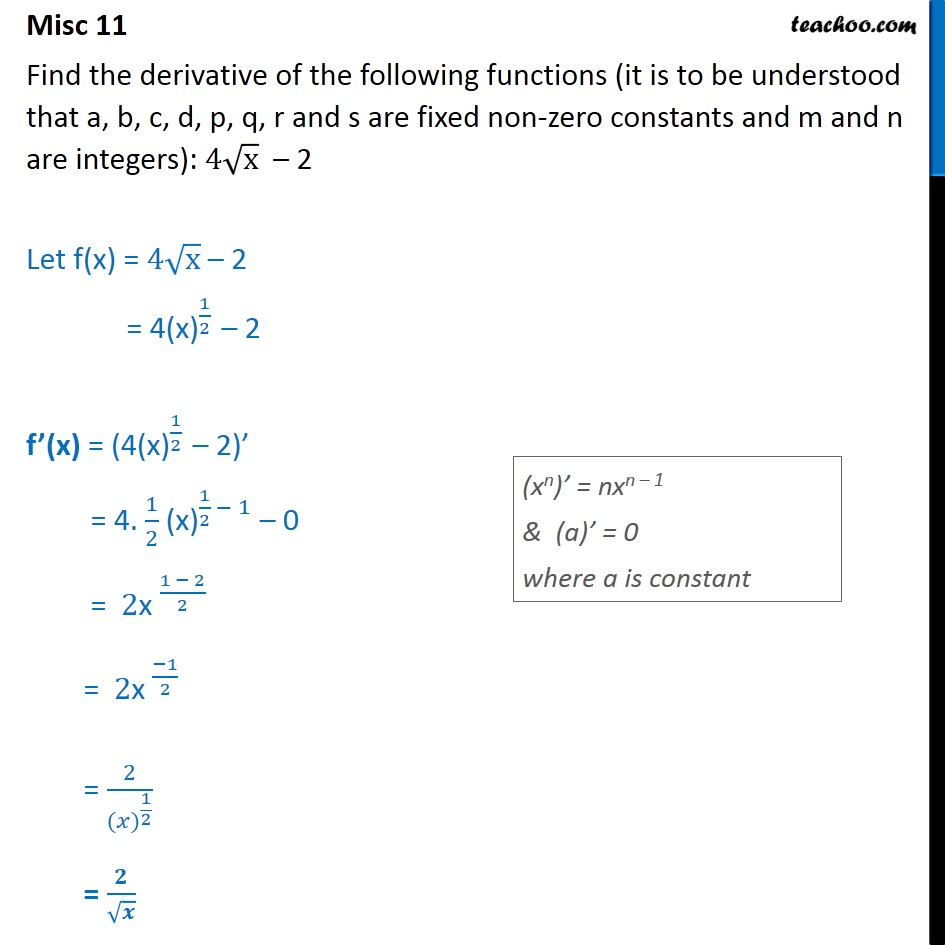Miscellaneous

Chapter 12 Class 11 Limits and Derivatives
Serial order wiseLearn in your speed, with individual attention - Teachoo Maths 1-on-1 Class

### Transcript

Misc 11 Find the derivative of the following functions (it is to be understood that a, b, c, d, p, q, r and s are fixed non-zero constants and m and n are integers): 4 x 2 Let f(x) = 4 x 2 = 4(x) 1 2 2 f (x) = ( 4(x) 1 2 2) = 4. 1 2 (x) 1 2 1 0 = 2x 1 2 2 = 2x 1 2 = 2 ( ) 1 2 =